slider_17slider_6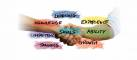slider_14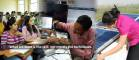slider_7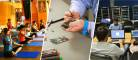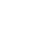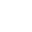## Certificate in Vedic Maths

Duration : 240 Hrs

Vedic Mathematics is a collection of Techniques/Sutras to solve mathematical arithmetics in easy and faster way. It consists of 16 Sutras (Formulae) and 13 sub-sutras (Sub Formulae) which can be used for problems involved in arithmetic, algebra, geometry, calculus, conics.

Veda is a Sanskrit word which means ‘Knowledge’.

Using regular mathematical steps, solving problems sometimes are complex and time consuming. But using Vedic Mathematic’s General Techniques (applicable to all sets of given data) and Specific Techniques (applicable to specific sets of given data), numerical calculations can be done very fast.

SCHEME OF EXAM

Theory
Minimum Maximum
16 40
External
Minimum Maximum
20 50
Internal
Minimum Maximum
4 10
Total Marks
Minimum Maximum
40 100
Minimum Maximum
C A+
ELIGIBILITY COURSE DURATION
Months Hours
Class VI 3 Month 240 Hrs
FEES
Total
2000